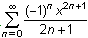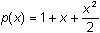Module 24 - Power Series Introduction | Lesson 1 | Lesson 2 | Lesson 3 | Self-Test Self Test The Maclaurin series for y = tan-1(x) is. What is the fifth-order Maclaurin polynomial for y = tan-1 (x)? Graph y = tan-1 (x) with a thick graphing style. In the same window graph the 5th-, 9th-, and 13th-order Maclaurin polynomials for this function. Estimate the interval of convergence for the series based on your graph. Find the second-order Taylor polynomial centered at 2 for y = ex. The second order Maclaurin polynomial for f(x) = ex is. Use this series to find the fourth-order Maclaurin polynomial for y = ex2 . Graph y = ex2 with a thick graphing style together with the fourth-order Maclaurin polynomial for y = ex2. Click here to check your answers. < Back ©Copyright 2007 All rights reserved. | Trademarks | Privacy Policy | Link Policy